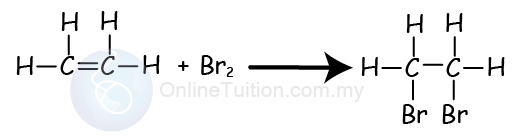# Chemical Properties of Alkenes

## Chemical Properties of Alkenes

1. Alkenes are chemically more reactive than alkanes.
2. This because alkenes are unsaturated hydrocarbons that have a double bond, C=C, between two carbon atom.
3. Almost all of the chemical reactions of alkene occur at the double bond.
4. Alkenes can undergo:
1. Combustion reaction
3. Polymerisation reaction

### Combustion of Alkenes

1. Like any other hydrocarbons, alkenes burn in air or oxygen.
2. Like alkanes,
1. alkene burns entirely in the sufficient oxygen to produce carbon dioxide and water.
2. alkene burns incompletely in a limited supply of oxygen to produce carbon monoxide, carbon (in the form of soot) and water.

Examples
Complete combustion of ethene

C2H4 + 3O2 → 2CO2 + 2H2O

Incomplete combustion ethene

C2H4 + 2O2 → 2CO + 2H2O
C2H4 + O2 → 2C + 2H2O

The combustion of alkenes becomes more difficult and produces more soot when the size of the alkenes molecules increases.

##### Percentage of Carbon

Example
Find and compare the percentage of carbon of ethene, propene and butene. [Relative atomic mass: C = 12, H = 1]
Percentage of carbon of ethene (C2H4)
$\begin{gathered} {\text{Percentage of Carbon}} \hfill \\ {\text{ = }}\frac{{2\left( {{\text{12}}} \right)}}{{2\left( {{\text{12}}} \right){\text{ + 4}}\left( 1 \right)}} \times 100\% \hfill \\ = 85.7\% \hfill \\ \end{gathered}$
Percentage of carbon of propene (C3H6)
$\begin{gathered} {\text{Percentage of Carbon}} \hfill \\ {\text{ = }}\frac{{3\left( {{\text{12}}} \right)}}{{3\left( {{\text{12}}} \right){\text{ + 6}}\left( 1 \right)}} \times 100\% \hfill \\ = 85.7\% \hfill \\ \end{gathered}$
Percentage of carbon of butene (C4H8)
$\begin{gathered} {\text{Percentage of Carbon}} \hfill \\ {\text{ = }}\frac{{4\left( {{\text{12}}} \right)}}{{4\left( {{\text{12}}} \right){\text{ + 8}}\left( 1 \right)}} \times 100\% \hfill \\ = 85.7\% \hfill \\ \end{gathered}$
Note::
All alkenes have the same percentage of carbon.

Example
Find and compare the percentage of carbon of hexane and hexene.
[Relative atomic mass: C = 12, H = 1]
Percentage of carbon of hexane (C6H14)
$\begin{gathered} {\text{Percentage of Carbon}} \hfill \\ {\text{ = }}\frac{{6\left( {{\text{12}}} \right)}}{{6\left( {{\text{12}}} \right){\text{ + 14}}\left( 1 \right)}} \times 100\% \hfill \\ = 83.7\% \hfill \\ \end{gathered}$
Percentage of carbon of hexene (C6H12) = 85.7% (Refer to example above)

Note:

1. Percentage of carbon of alkene is higher compare to the alkane that has same number of carbon per molecule.
2. Therefore, combustion of alkene will produce more sooty flame compared to their corresponding alkane.
3. Alkenes have a higher percentage of carbon compared to their corresponding alkane.
1. Alkenes are unsaturated hydrocarbon, hence they undergo addition reactions.
2. Addition reaction occurs when other atoms are added to each carbon atom of the double bond, –C=C– to form a single covalent bond.

Example,

Alkenes may undergo the addition reaction with
1. halogen
2. steam
3. hydrogen
4. halogen halide
5. potassium permanganate(VII)

### Hydrogenation of Alkenes

1. Alkene can undergo reaction with hydrogen gas.
2. The process is also called hydrogenation.

Equation:

### Note:

1. This reaction converts unsaturated hydrocarbon to saturated hydrocarbon.
2. The condition for the reaction:
1. Catalyst: Platinum/nickel
2. Temperature: 180ºC
3. This is the process used to produce margarine.
##### The reaction of Alkenes with Hydrogen Halide

Alkenes react with hydrogen halides (such as hydrogen chloride, hydrogen bromide and hydrogen iodide) at room temperature to produce saturated organic compounds called halogenoalkanes.

#### Ethene React with Hydrogen Chloride

(Ethene react with hydrogen chloride produces chloroethane.)

#### Ethene React with Hydrogen Bromide

(Ethene react with hydrogen bromide produces bromoethane.)

#### Ethene React with Hydrogen Iodide

(Ethene react with hydrogen iodide produces iodoethane.)
##### Halogenation of Alkenes
1. Alkenes undergo the addition reaction with a halogen such as fluorine, chlorine, bromine and iodine.
2. Since this is the reaction with the halogens, hence it is also called the halogenation reaction.
3. The equation below shows the addition reaction between ethene and bromine.1. When ethane gas is bubbled into bromine liquid, the brown colour bromine turns colourless.
2. The product produced is 1,2-dibromoethane.
3. If chlorine gas is used instead of bromine, the yellow colour of chlorine water will be decolourised.
##### Hydration of Alkenes
1. Alkene can react with steam (water) to produce alcohol.
2. This process is an addition reaction.
3. It’s also called the hydration reaction.
4. The equation below shows the addition reaction of ethene with steam.1. The alkene is converted to become alcohol
2. The condition of the reaction is as below:
1. Catalyst: Phosphoric acid
2. Temperature: 300ºC
3. Pressure: 60 atm
3. Alcohol can be changed back to alkenes through the dehydration reaction.
##### Oxidation of Alkenes
1. Alkenes can also react with oxidising agents such as potassium permanganate(VII) to produce a diol.
2. A diol is an alcohol with 2 hydroxyl group (-OH).
3. This process is an addition reaction.
4. Chemical reaction below shows the oxidation reaction (also addition reaction) between ethene and potassium permanganate(VII).1. In the reaction, we use the square bracket with an O, [O], to represent the oxidising agent.
2. The product produced is ethan-1,2-diol.
3. During the reaction, the purple colour of acidified potassium manganate (VII) solution change to colourless.